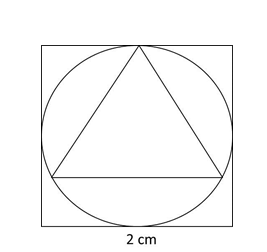# CAT Quantitative Aptitude Questions | Geometry Questions for CAT - Triangles

###### CAT Questions | CAT Geometry Questions | Circle, Square and Triangle

The question is from CAT Geometry - Triangles. In this problem it discusses about the relation between the sides of square , triangle and the radius of the circle. CAT Geometry questions are heavily tested in CAT exam. Make sure you master Geometry problems.

Question 22: A circle inscribed in a square of side 2 has an equilateral triangle inscribed inside it. What is the ratio of areas of the equilateral triangle to that of the square?

1. 9√3 : 16
2. 3√3 : 4
3. 9√3 : 4
4. 3√3 : 16

## Best CAT Coaching in Chennai

#### CAT Coaching in Chennai - CAT 2022Limited Seats Available - Register Now!If we considered the side of the equilateral triangle to be ‘a’, the circumradius of an equilateral triangle is R = $$frac{a}{√3}\\$ Therefore, a = √3 So, ratio of areas of the equilateral triangle to that of the square is $\frac{√3}{4}\\$ *$√3)2 : 22